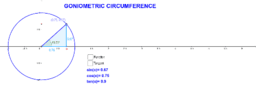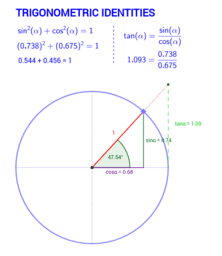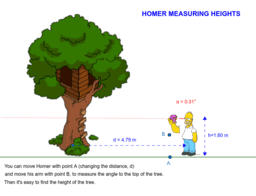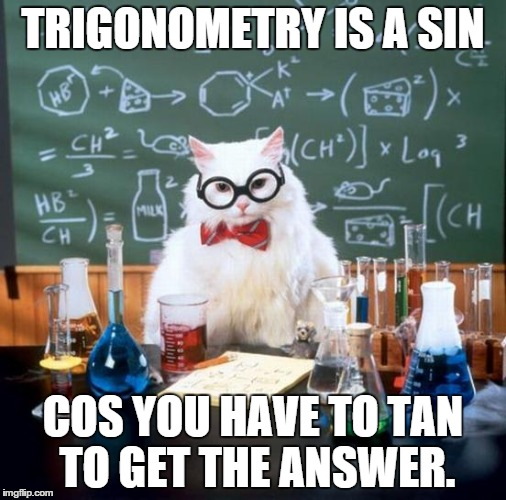# Mates y TIC - Maths and ICT

## Actividades de Matemáticas con TIC - Math Activities with ICT - - - (matesytic@gmail.com) Ricardo García Mesa

•# Archive for January, 2019

## Moving Homer with vectors

Posted by ricardogm on 31st January 2019

Hi

We are going to use vectors to create animations, similar to this applet:Pay attention and send me the files, as usual.

The math thing we are going to use is “linear combinations of vectors“, which is simply a·u+b·v, being  u and v vectors and a and b, numbers.

1. First, an easier construction. We are going to generate something similar to this:2. Now, the Homer thing. It’s more difficult, so you have to pay even more attention than usual.

The images you need are in this folder:

Images

Posted in 4º ESO | No Comments »

## Problemas de optimización resueltos

Posted by ricardogm on 29th January 2019

Pues eso:

Problemas

## Pasos para la resolución de problemas de optimización:

1  Se plantea la función que hay que maximizar o minimizar.

2  Se plantea una ecuación que relacione las distintas variables del problema, en el caso de que haya más de una variable.

3  Se despeja una variable de la ecuación y se sustituye en la función de modo que nos quede una sola variable.

4  Se deriva la función y se iguala a cero, para hallar los máximos-mínimos locales.

5  Se realiza la 2ª derivada para comprobar el resultado obtenido o, alternativamente, se comprueba el valor de la función en los máximos-mínimos y en los extremos de su dominio.

Posted in 2º Bach. CT | No Comments »

## Trigonometric ratios in every quadrant

Posted by ricardogm on 28th January 2019

Hi

We have seen this thing before:Now we are going to work with it a little more. Go to this page.

Posted in 4º ESO | No Comments »

## Trigonometric identities and homer measuring trees

Posted by ricardogm on 21st January 2019

Hi

We are going to prove these two famous trigonometic identities:

sin2(x) + cos2(x) = 1

tan(x)=cos(x)/sin(x)

that can be seen in this applet:​And how to measure trees with trigonometry (we are going to do more or less the same thing):Posted in 4º ESO | No Comments »

## Goniometric circumference

Posted by ricardogm on 18th January 2019Hi

Today we are going to create something similar to this:The idea is to study the trigonometric ratios of angles greater than 90º.

Pay attention. Send me the file.

Now time to solve some problems in your notebook:

trigonometry-word-problems.pdfPosted in 4º ESO | No Comments »

## Soluciones temas 13 y 14

Posted by ricardogm on 18th January 2019Pues eso:

Tema 13

Tema 14

Posted in 2º Bach. CT | No Comments »

## 3-gonometry-2

Posted by ricardogm on 10th January 2019Hi

We have some things to do:

0. Solve these problems in your notebook while I try to correct your homework. Make drawings, of course.

1 The known data for a right triangle ABC is a = 5 m and B = 41.7°. Solve the triangle.

2 The known data for a right triangle ABC is b = 3 m and B = 54.6°. Solve the triangle.

3 The known data for a right triangle ABC is a = 6 m and b = 4 m. Solve the triangle.

4 The known data for a right triangle ABC is b = 3 m and c = 5 m. Solve the triangle.

5 A tree 50 m tall casts a shadow 60 m long. Find the angle of elevation of the sun at that time.

6 An airship is flying at an altitude of 800 m when it spots a village in the distance with a depression angle of 12°. How far is the village from where the plane is flying over?

7 Find the radius of a circle knowing that a chord of 24.6 m has a corresponding arc of 70°.

8 Calculate the area of a triangular field, knowing that two of its sides measure 80 m and 130 m and between them is an angle of 70°.

9 Calculate the height of a tree, knowing that from a point on the ground the top of the tree can be seen at an angle of 30º and from 10 m closer the top can be seen at an angle of 60°.

10 The length of the side of a regular octagon is 12 m. Find the radii of the inscribed and circumscribed circles.

11 Calculate the length of the side and the apothem of a regular octagon inscribed in a circle with a radius of 49 centimeters.

12 Three towns A, B and C are connected by roads which form a triangle. The distance from A to C is 6 km and from B to C, 9 km. The angle between these roads is 120°. How far are the towns A and B from each other?

2. More problems.

The drawing below shows nicely what we are going to do:Posted in 4º ESO | No Comments »